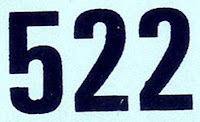## Wednesday, October 20, 2010

### 522

522 = 2 x 32 x 29.

522 has a representation as a sum of two squares: 522 = 92 + 212.

522 is the sum of six consecutive primes: 522 = 73 + 79 + 83 + 898 + 97 + 101.

522 is the sum of seven positive fifth powers: 522 = 15 + 15 + 15 + 15 + 25 + 35 + 35.The SOKO 522 was a two-seater, Yugoslavian military training and light attack aircraft produced in the 1950s.

Source: Wikipedia